# 1. Intro¶

In this project we'll build a machine learning model to predict the amount of bicycles rented every day in Washington D.C. in 2011 and 2012. There are a number of factors that influence the number of bike rents every day, very often those factors influence each other. A linear regression model is probably not going to deliver any good results in this scenario.

We'll start with simple linear regression model, just to confirm the hunch that it won't perform well. Then we'll move on to Random Forest Regressor and perform basic GridSearch to tweak that model. After that we'll test other models, including gradient boosting models and neural networks. Having tested quite a few models we'll move on to joining them together:

• why rely on single model when we can use 2 or more models to predict the rental values, then calculate the average of predicted value?
• we'll also try to build a meta model that learns on the output from other machine learning algorithms

Index:# 1.1. What is bicycle sharing?¶

In :
!pip install wikipedia
import wikipedia
import textwrap as tr

Collecting wikipedia
Requirement already satisfied: beautifulsoup4 in /opt/conda/lib/python3.7/site-packages (from wikipedia) (4.10.0)
Requirement already satisfied: requests<3.0.0,>=2.0.0 in /opt/conda/lib/python3.7/site-packages (from wikipedia) (2.25.1)
Requirement already satisfied: certifi>=2017.4.17 in /opt/conda/lib/python3.7/site-packages (from requests<3.0.0,>=2.0.0->wikipedia) (2021.10.8)
Requirement already satisfied: urllib3<1.27,>=1.21.1 in /opt/conda/lib/python3.7/site-packages (from requests<3.0.0,>=2.0.0->wikipedia) (1.26.6)
Requirement already satisfied: chardet<5,>=3.0.2 in /opt/conda/lib/python3.7/site-packages (from requests<3.0.0,>=2.0.0->wikipedia) (4.0.0)
Requirement already satisfied: idna<3,>=2.5 in /opt/conda/lib/python3.7/site-packages (from requests<3.0.0,>=2.0.0->wikipedia) (2.10)
Requirement already satisfied: soupsieve>1.2 in /opt/conda/lib/python3.7/site-packages (from beautifulsoup4->wikipedia) (2.2.1)
Building wheels for collected packages: wikipedia
Building wheel for wikipedia (setup.py) ... - \ done
Created wheel for wikipedia: filename=wikipedia-1.4.0-py3-none-any.whl size=11696 sha256=7e3a7d31d8cc7cb01e7180e83315f72211f29b9852cf2e6fe957bf54f2b552cf
Stored in directory: /root/.cache/pip/wheels/15/93/6d/5b2c68b8a64c7a7a04947b4ed6d89fb557dcc6bc27d1d7f3ba
Successfully built wikipedia
Installing collected packages: wikipedia
Successfully installed wikipedia-1.4.0
WARNING: Running pip as the 'root' user can result in broken permissions and conflicting behaviour with the system package manager. It is recommended to use a virtual environment instead: https://pip.pypa.io/warnings/venv

In :
my_str = wikipedia.summary("Bicycle-sharing system")
for line in tr.wrap(my_str, width=110):
print(line)
print('source: Wikipedia')

A bicycle-sharing system, bike share program, public bicycle scheme, or public bike share (PBS) scheme, is a
shared transport service in which bicycles are made available for shared use to individuals on a short term
basis for a price or free. Many bike share systems allow people to borrow a bike from a "dock" and return it
at another dock belonging to the same system. Docks are special bike racks that lock the bike, and only
release it by computer control. The user enters payment information, and the computer unlocks a bike. The user
returns the bike by placing it in the dock, which locks it in place. Other systems are dockless. In recent
years, an increasing number of cities across the world have started to offer both mechanical bike share and
electric bicycle sharing systems, such as Dubai, New York, Paris, Montreal and Barcelona.For many systems,
smartphone mapping apps show nearby available bikes and open docks. In July 2020, Google Maps began including
bike shares in its route recommendations.
source: Wikipedia


# 1.2 Importing packages¶

In :
import pandas as pd
import numpy as np
import random
import seaborn as sns
import matplotlib.pyplot as plt
from sklearn.model_selection import train_test_split
from sklearn.preprocessing import StandardScaler, OrdinalEncoder
# from sklearn.impute import SimpleImputer
# from sklearn.compose import ColumnTransformer
from sklearn import linear_model
from sklearn.ensemble import RandomForestRegressor
from sklearn.metrics import r2_score
from sklearn.metrics import mean_squared_error
from sklearn.preprocessing import OneHotEncoder
from sklearn.model_selection import GridSearchCV
from sklearn.preprocessing import MinMaxScaler
import missingno as msno


# 2.1. Initial Analysis¶

In :
df = pd.read_csv('../input/bike-sharing-dataset/hour.csv')

Out:
instant dteday season yr mnth hr holiday weekday workingday weathersit temp atemp hum windspeed casual registered cnt
0 1 2011-01-01 1 0 1 0 0 6 0 1 0.24 0.2879 0.81 0.0 3 13 16
1 2 2011-01-01 1 0 1 1 0 6 0 1 0.22 0.2727 0.80 0.0 8 32 40
2 3 2011-01-01 1 0 1 2 0 6 0 1 0.22 0.2727 0.80 0.0 5 27 32
3 4 2011-01-01 1 0 1 3 0 6 0 1 0.24 0.2879 0.75 0.0 3 10 13
4 5 2011-01-01 1 0 1 4 0 6 0 1 0.24 0.2879 0.75 0.0 0 1 1
In :
df.isnull().sum().sum()

Out:
0
In :
df.describe().T

Out:
count mean std min 25% 50% 75% max
instant 17379.0 8690.000000 5017.029500 1.00 4345.5000 8690.0000 13034.5000 17379.0000
season 17379.0 2.501640 1.106918 1.00 2.0000 3.0000 3.0000 4.0000
yr 17379.0 0.502561 0.500008 0.00 0.0000 1.0000 1.0000 1.0000
mnth 17379.0 6.537775 3.438776 1.00 4.0000 7.0000 10.0000 12.0000
hr 17379.0 11.546752 6.914405 0.00 6.0000 12.0000 18.0000 23.0000
holiday 17379.0 0.028770 0.167165 0.00 0.0000 0.0000 0.0000 1.0000
weekday 17379.0 3.003683 2.005771 0.00 1.0000 3.0000 5.0000 6.0000
workingday 17379.0 0.682721 0.465431 0.00 0.0000 1.0000 1.0000 1.0000
weathersit 17379.0 1.425283 0.639357 1.00 1.0000 1.0000 2.0000 4.0000
temp 17379.0 0.496987 0.192556 0.02 0.3400 0.5000 0.6600 1.0000
atemp 17379.0 0.475775 0.171850 0.00 0.3333 0.4848 0.6212 1.0000
hum 17379.0 0.627229 0.192930 0.00 0.4800 0.6300 0.7800 1.0000
windspeed 17379.0 0.190098 0.122340 0.00 0.1045 0.1940 0.2537 0.8507
casual 17379.0 35.676218 49.305030 0.00 4.0000 17.0000 48.0000 367.0000
registered 17379.0 153.786869 151.357286 0.00 34.0000 115.0000 220.0000 886.0000
cnt 17379.0 189.463088 181.387599 1.00 40.0000 142.0000 281.0000 977.0000

### Windspeed is not normalized¶

In :
# lets generate a correlation heatmap:
fig, ax = plt.subplots(figsize=(16,16))
plt.show()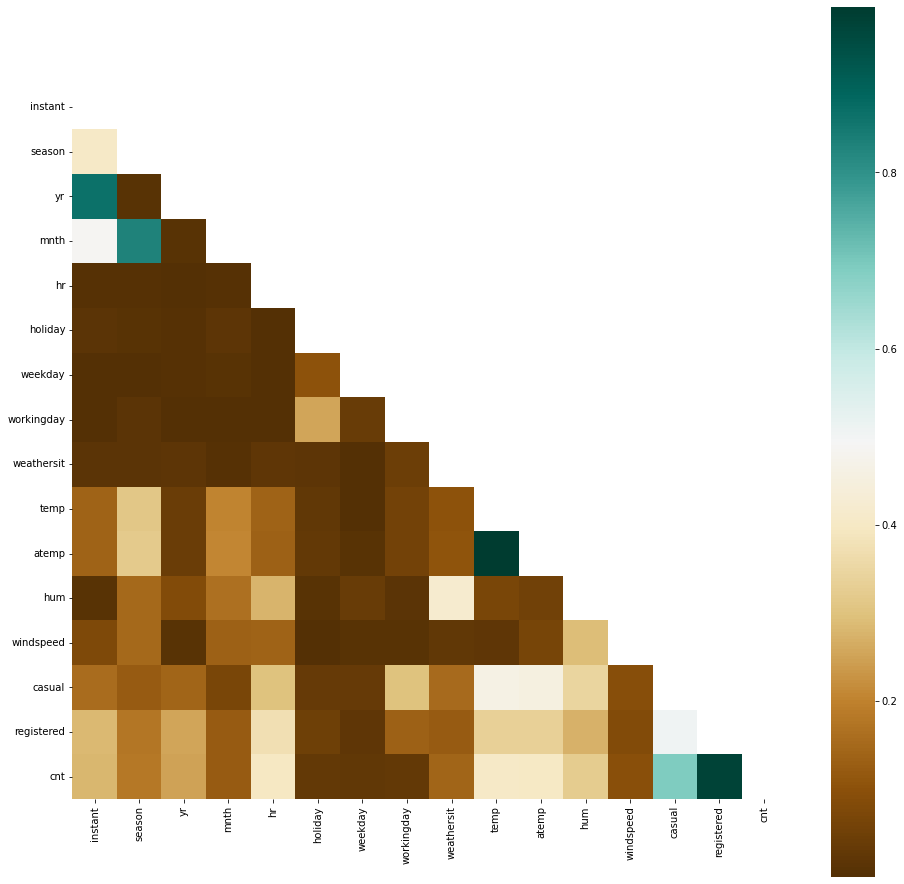In :
# we'll use the usual spines function to reduce plots code:
def spines(ax,yl='Rental counts',xl='',title=''):
x1 = ax.spines['right'].set_visible(False)
x2 = ax.spines['top'].set_visible(False)
x3 = ax.spines['left'].set_linewidth(2)
x4 = ax.spines['bottom'].set_linewidth(2)
x5 = ax.set_ylabel(yl)
x6 = ax.set_xlabel(xl)
x7 = ax.set_title(title)
return x1, x2, x3, x4, x5, x6

fig, ax = plt.subplots(figsize=(16,16))
ax = plt.subplot(221)
plt.pie([df['registered'].sum(), df['casual'].sum()], labels=['registered','casual'])

ax = plt.subplot(222)
df.groupby('hr')['cnt'].sum().plot.bar()
spines(ax, title='Combined')

ax = plt.subplot(223)
df.groupby('hr')['registered'].sum().plot.bar()
spines(ax, title='Registered')

ax = plt.subplot(224)
df.groupby('hr')['casual'].sum().plot.bar()
spines(ax, title='casual')

plt.show()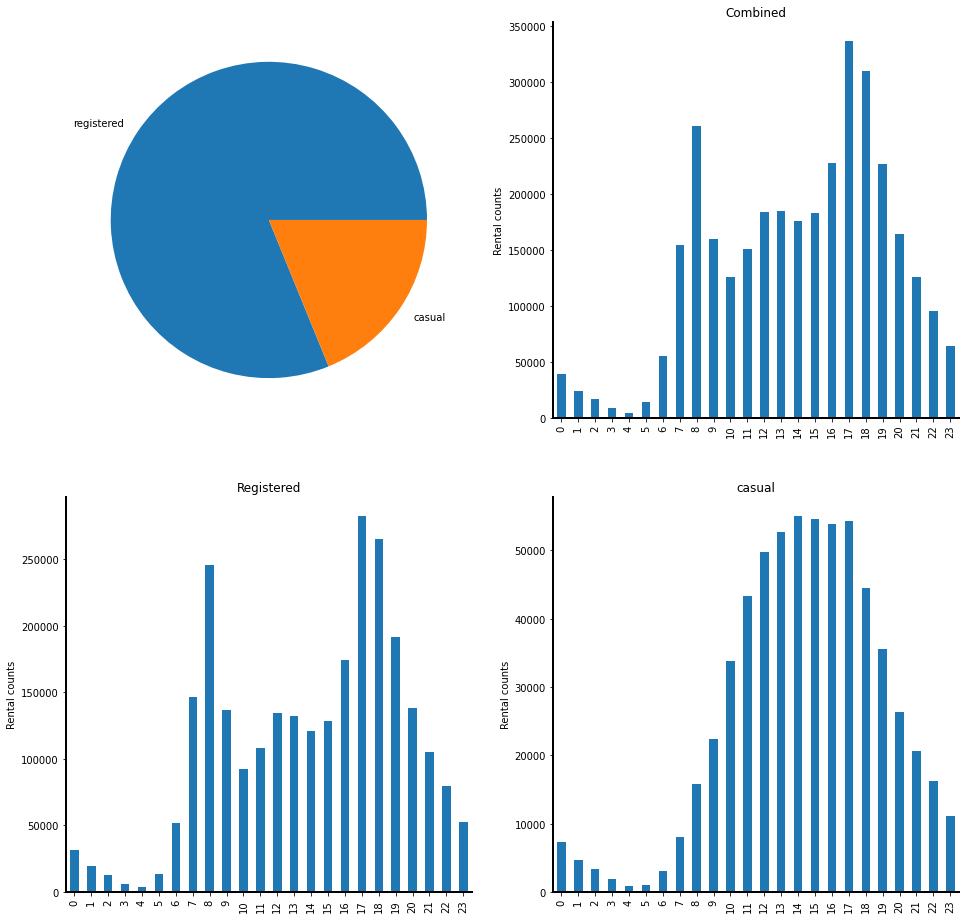Observations:

• registered users are the vast majority of rental users
• unfortunately their rental count is distributed throughout the day in a much more complex way than casual users rentals

# 2.2. Time columns:¶

In :
fig, ax = plt.subplots(figsize=(16,8))
sns.stripplot(x = df.groupby('dteday')['cnt'].sum().index, y = df.groupby('dteday')['cnt'].sum(), alpha=0.3, color='black', size=6, jitter=0.4, zorder=2)
spines(ax, title='Counts per date', xl='Date')
X_TICKS = 50
plt.xticks(range(0, len(df['dteday'].sort_values().unique()), X_TICKS), df['dteday'].sort_values().unique()[::X_TICKS], rotation = 30)
plt.show()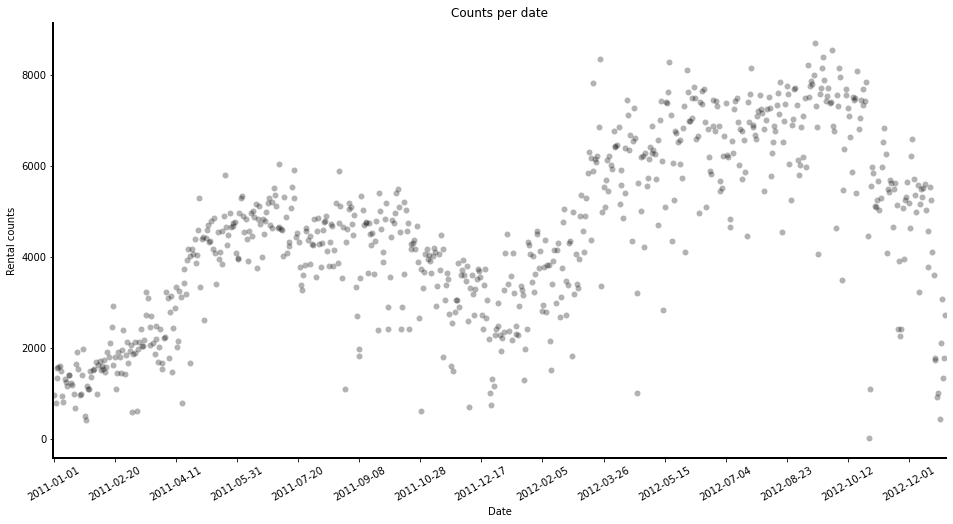In :
fig, ax = plt.subplots(figsize=(16,8))
sns.stripplot(x = df['hr'], y = df['cnt'], alpha=0.3, color='black', size=6, jitter=0.4, zorder=2)
plt.plot(df['hr'].unique(),df.groupby('hr')['cnt'].mean(), color='red', linewidth=6, zorder=3, alpha=0.6)
plt.legend(['avg','counts'])
spines(ax, xl='Hour', title='Rental counts per hour')
plt.show()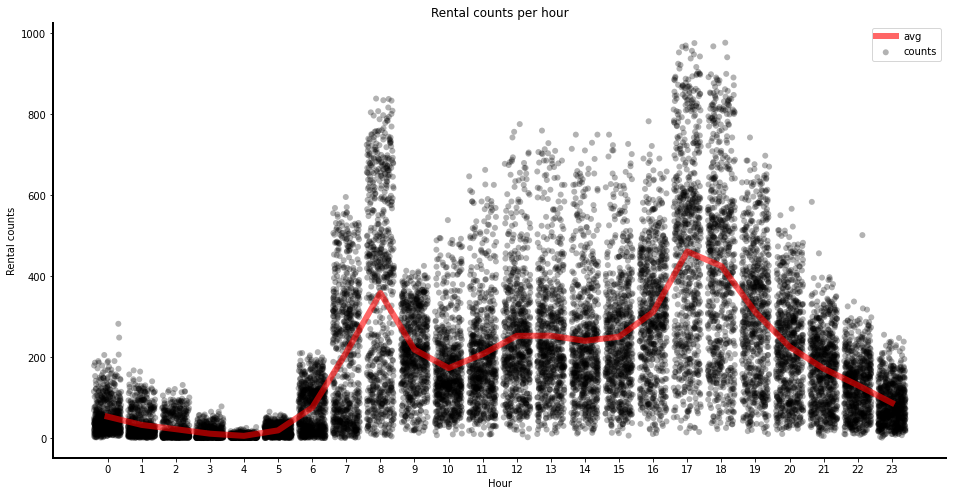In :
fig, ax = plt.subplots(figsize=(16,8))
sns.stripplot(x = df['weekday'], y = df['cnt'], alpha=0.3, color='black', size=6, jitter=0.4, zorder=2)
plt.plot(df['weekday'].sort_values().unique(),df.groupby('weekday')['cnt'].mean(), color='red', linewidth=6, zorder=3, alpha=0.6)
plt.legend(['avg','counts'])
spines(ax, xl='day',  title='Rental counts per day')
plt.show()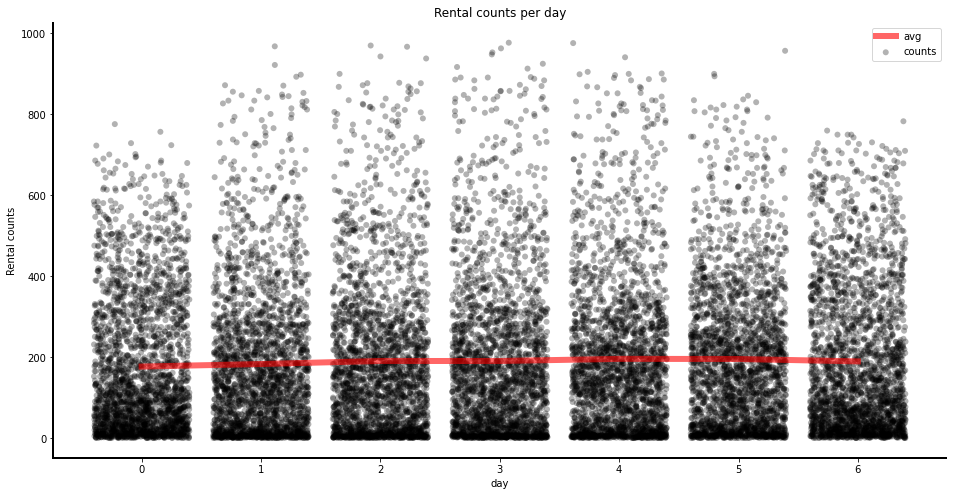In :
fig, ax = plt.subplots(figsize=(16,8))
sns.stripplot(x = df['mnth'], y = df['cnt'], alpha=0.3, color='black', size=6, jitter=0.4, zorder=2)
plt.plot(df['mnth'].unique()-1,df.groupby('mnth')['cnt'].mean(), color='red', linewidth=6, zorder=3, alpha=0.6)
# handles, labels = ax.get_legend_handles_labels()
plt.legend(['avg','counts'])
spines(ax, xl='mnth',  title='Rental counts per month')
plt.show()